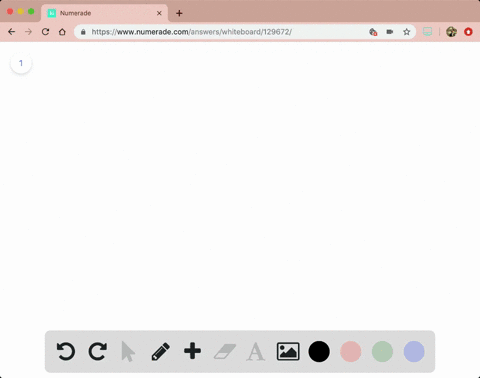Enroll in one of our FREE online STEM bootcamps. Join today and start acing your classes!View Bootcamps### a. Find the open intervals on which the function …

03:51University of California, Riverside
Problem 39

# a. Find the open intervals on which the function is increasing and decreasing.b. Identify the function's local and absolute extreme values, if any, saying where they occur.$$h(x)=x^{1 / 3}\left(x^{2}-4\right)$$

## Discussion

You must be signed in to discuss.

## Video Transcript

Okay, so four problem 21. Um, th acts. The function given to us is, um, elective. Say Ajax is defined to be negative, x cubed plus two x squared. And if we take the real tip off this function, we can find it is 93 x squared plus or axe. And that is to be positive. Then we have, um, take out a takeout acts we have next three acts plus four bigger than zero. So this will be a poor Abla with solution one. Sorry. Zero and or third, I should be looked like this. Okay, so that means decision to this inequality will be X belongs to 0 to 4/3. So on this interval, function will be increasing. So, um, that means from negative infinity to zero union or third to infinity function will be decreasing. Okay. Now, for part R. B, we need to find the local extreme and absolute extreme. So we're this problem if you check the locally screen, it's definitely f zero and have or third, but no off. These are absolute extreme, because the function will look like, um, should we look like this? Where at zero, we have a local extreme and at for third, we have another locally stream. But there is no absolute absolute maximum an absolute minimum, so no absolute extreme.

#### You're viewing a similar answer. To request the exact answer, fill out the form below:

Our educator team will work on creating an answer for you in the next 6 hours.